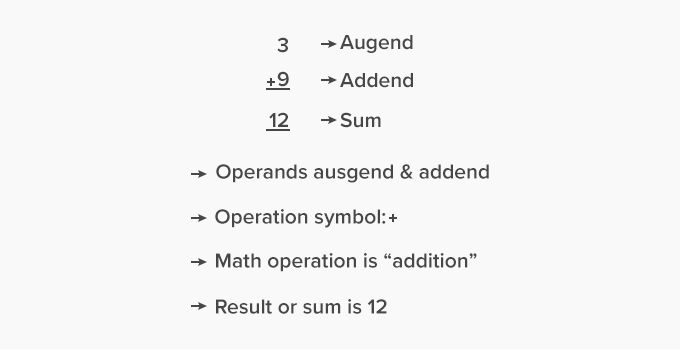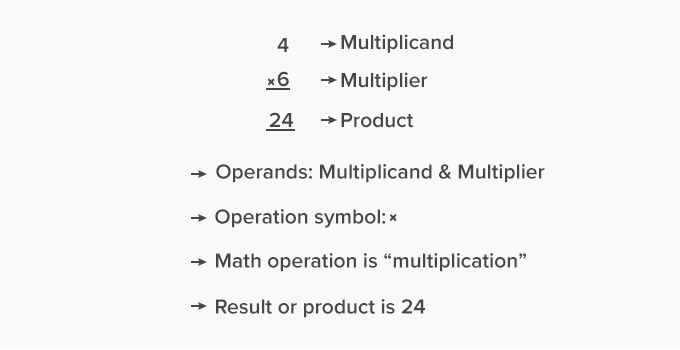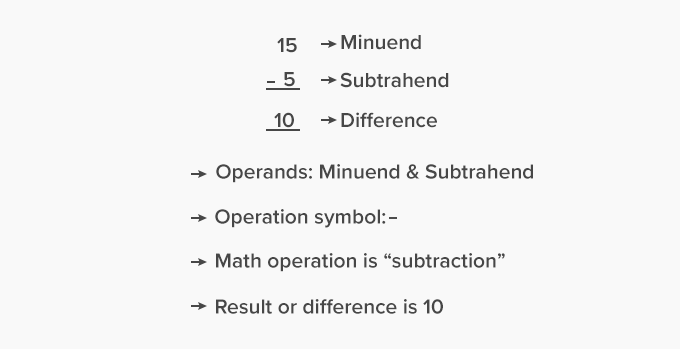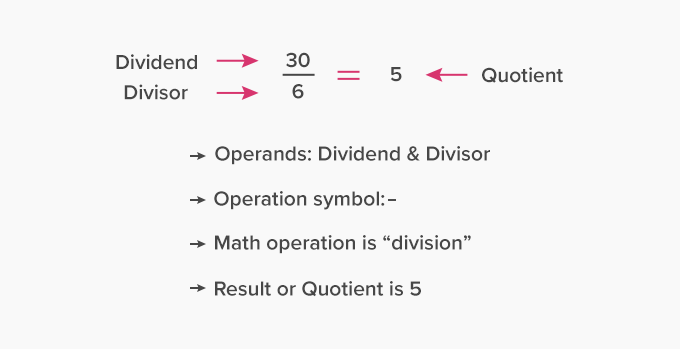# Operation - Definition with Examples

The Complete K-5 Math Learning Program Built for Your Child

• 30 Million Kids

Loved by kids and parent worldwide

• 50,000 Schools

Trusted by teachers across schools

• Comprehensive Curriculum

Aligned to Common Core

## Operation

The mathematical “operation” refers to calculating a value using operands and a math operator. The symbol of the math operator has predefined rules to be applied to the given operands or numbers.Image: The symbols of basic math operations (id=646579018)

A mathematical expression is a set of numbers and operations. The elements of a math expression performing a math operation are:Operands – The numbers used for an operation are called operands. Based on the type of operation, different terms are assigned to the operands.

Operator – The symbol indicating a math operation is an operator, for example:

• − for subtraction
• × for multiplication
• ÷ for division
•  = for equal to, indicates the equivalence, that is the left hand side value is equal to the right hand side value.

The table below shows the basic math operations along with the terms assigned to operands and the resultant value.To understand the utility of math operations listed above, let us consider another example.

Sarah has two baskets full of fruits. The first basket has 5 apples and the second basket has 6 bananas. She mixes them in a big basket. How many fruits are there in the big basket?Image: Fruit baskets with apples and bananas (id = 386628463, 1205236333, 397222102)

• First basket = 5 apples
• Second basket = 6 bananas
• Total fruits in the big basket = 5 apples and 6 bananas
• Thus, the math expression with operands 5 and 6 and operator “+” will be:
• Total fruits = 5 + 6 = 11
• Total fruits = 11

The basic operations (addition and subtraction) are used in different forms such as multiplication (repeated addition) and division (repeated subtraction).  Using these operations, the word problems are modeled into math expressions.

 Fun facts The historic term for math operations is “hesab” from the Arabian scriptures The first calculating device performing basic math operations was “Abacus”

Related math vocabulary

• Math operations
• Operators
• Math expression
Won Numerous Awards & Honors